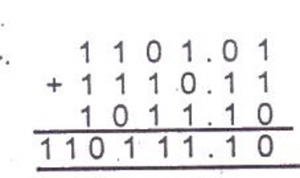# Mathematics 2017 Past Questions | JAMB

Study the following Mathematics past questions and answers for JAMBWAEC  NECO and Post-JAMB. Get prepared with official past questions and answers for upcoming examinations.

11.  Simplify 3 $$^{n − 1}$$ ×  $$\frac{27^{n + 1}}{81^n}$$

• A. 3$$^{2n}$$
• B. 9
• C. 3n
• D. 3 $$^{n + 1}$$

12. The locus of a point which is equidistant from the line PQ forms a

• A. circle centre P
• B. pair of parallel lines each opposite to PQ
• C. circle centre Q
• D. perpendicular line to PQ

13.  Given T = {even numbers from 1 to 12}

N = {common factors of 6, 8 and 12} Find T n N

• A. {2, 3}
• B. {2, 3, 4}
• C. {3, 4, 6}
• D. {2}

14.Given the quadrilateral RSTO inscribed in the circle with O as centre. Find the size angle x and given RST = 60o
• A. 100o
• B. 140o
• C. 120o
• D. 10o

15. Find the sum of the range and the mode of the set of numbers 10, 9, 10, 9, 8, 7, 7, 10, 8, 10, 8, 4, 6, 9, 10, 9, 7, 10, 6, 5

• A. 16
• B. 14
• C. 12
• D. 10

16.  Find the sum to infinity of the series $$\frac{1}{4}$$, $$\frac{1}{8}$$, $$\frac{1}{16}$$,……….

• A. $$\frac{1}{2}$$
• B. $$\frac{3}{5}$$
• C. $$\frac{-1}{5}$$
• D. $$\frac{73}{12}$$

17.The base in which the operation was performed was
• A. 6
• B. 2
• C. 4
• D. 5

18. The value of x + x ( xx) when x = 2 is

• A. 16
• B. 10
• C. 18
• D. 24

19. In a regular polygon, each interior angle doubles its corresponding exterior angle. Find the number of sides of the polygon

• A. 8
• B. 6
• C. 4
• D. 3

20. A cylindrical tank has a capacity of 3080m3. What is the depth of the tank if the diameter of its base is 14m? Take pi = 22/7.

• A. 23m
• B. 25m
• C. 20m
• D. 22m
Subscribe
Notify of